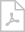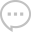# [SOLVED] Help for keypress event for decimal numbers

0
0

Howdy,

Someone helped mt for the keypress event, for just digits, but i’d like to ask some help on how to achieve more.

str = “var e = arguments; if (‘0123456789’.indexOf(e.getKeyIdentifier()) === -1 && e.getKeyIdentifier() != ‘Backspace’)e.stop();”

How have the keypress for:

• decimal numbers, ex: 1283,93, so when the user types “.” it only can allow it once, and translate it to “,”
• for only letters from A-Z

Here’s the code i had for the decimal numbers, and also for the letters only.

## letters only ##

If _e.KeyChar = Chr(Keys.Back) Then
Exit Sub
End If
If Not Char.IsLetter(_e.KeyChar) Then
_e.Handled = True
End If

## decimal numbers ##

If _e.KeyChar.ToString = “.” Then
_e.Handled = True
SendKeys.Send(“,”)
End If
‘ fix 1
If _e.KeyChar = Chr(Keys.Back) Then
Exit Sub
End If
Dim cont As Int32
Dim dec() As String
Try
dec = Split(_Sender.Text, “,”)
Catch ex As Exception
Exit Sub
End Try
‘_NumNegativo = True
If _NumNegativo Then
‘ isto nao deixa meter o sinal menos ‘a direita
If _Sender.text.ToString.Trim <> “” Then
If _e.KeyChar.ToString = “-” Then
_e.Handled = True
End If
End If
End If

If dec.Length > 1 Then
‘ fix 2
If dec(0).Trim = “” Then
cont = 0
Else
cont = dec(1).Length
End If
Else
cont = 0
End If
If Not Char.IsDigit(_e.KeyChar) And Not _e.KeyChar.Equals(Chr(Keys.Back)) And Not _e.KeyChar.ToString = “,” Then
_e.Handled = True
ElseIf _e.KeyChar.ToString = “,” AndAlso _Sender.Text.IndexOf(“,”) <> -1 Then
_e.Handled = True
ElseIf _e.KeyChar.ToString = “-” AndAlso _Sender.Text.IndexOf(“-“) <> -1 Then
_e.Handled = True
‘ o numero de decimais possiveis, vem do argumento, e por default sao 2
ElseIf cont = _NumDec Then
‘ fix 3
If dec.Length > 1 Then
If _Sender.SelectionStart <= _Sender.Text.IndexOf(“,”) Then
‘ ok, o cursor esta do lado esquerdo da virgula, entao nao faz nada
Else
If _Sender.SelectionStart > _Sender.Text.IndexOf(“,”) Then
If _Sender.SelectedText.Length = 0 Then
_e.Handled = True
End If
End If
End If
End If
End If

• You must to post comments
0
0

Jorge,

digging a bit deeper and also considering that such keyboard filtering should better be done on the client side
than on the server side, you could also try the following with your TextBox:

`InitScript = "this.getChildControl("textfield").setFilter(/\d/);"`

https:/regex101.com/

Best regards,
Frank

•Hi Frank, Maskedtextbox it’s great. Doing it on initscript, will prevent server load, and make it to the client side, correct? if yes it’s a good option! Thanks for all your help!
• You must to post comments
0
0

Jorge,

It´s a way better approach than handling keypress events on the server side:

Best regards
Frank

• You must to post comments
0
0

Frank,

To allow only digits in a textbox, i have this in the addeventhandler
var e = arguments; if (‘0123456789’.indexOf(e.getKeyIdentifier()) === -1 && e.getKeyIdentifier() != ‘Backspace’)e.stop();

But i’d like to have a code to allow only the following format: “12541,33”

And another one to allow only letters from A to Z

Would it be possible?

• You must to post comments
0
0

Jorge,

I am not sure, what you are trying to achieve and what is the question.
Please provide a complete test case even though I doubt that this is really a Wisej specific topic/question.

Best regards
Frank

• You must to post comments
Showing 4 results### Share### Share